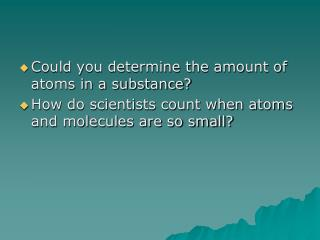DownloadDownload PresentationCould you determine the amount of atoms in a substance?

# Could you determine the amount of atoms in a substance?

Télécharger la présentation## Could you determine the amount of atoms in a substance?

- - - - - - - - - - - - - - - - - - - - - - - - - - - E N D - - - - - - - - - - - - - - - - - - - - - - - - - - -
##### Presentation Transcript

1. Could you determine the amount of atoms in a substance? How do scientists count when atoms and molecules are so small?

2. Using the concept of counting by weight Could you determine the amount of atoms in a piece of copper?

3. What information would you need? The periodic table has a secret! It can tell you the amount of atoms in a specific mass of each element

4. Look at the amu of copper. The amu also describes the number of atoms in a specific mass in g • Ex: There are 6.02 X10 23 atoms/63.546 g of copper • Could you determine the amount of atoms in any piece of Copper? • Go ahead…..

5. 6.02 X10 23 is a huge number!!! • It is a unit of measurement that scientists use. Just as chefs use the dozen (12) • This is manageable because atoms are so tiny • They call this number a mole!! (sometimes abbreviated mol) • With this new information could you determine the amount of moles if I gave you a specific mass of an element? • Write a conversion you could use. • Ex If I have .500g Al 1mol/26.98g How many mols do I have try it… • .500g Al 1 mol/26.98g = .0185 mols of Al

6. If I gave you the amount of mols could you give me the mass? • .496 mols of Al 26.98g Al/1 mol = 13.4 g Al • If I gave you the number of atoms could you determine the mass? • Remember there are 6.02 X10 23 atoms in a mol and the molar mass is the mass of one mole • Find the mass of 2.03 X10 23 atoms of S • 2.03 X10 23 atoms S 1 mol/6.02 X 10 23 atoms S 32.055g S/1 mol • Answer = 10.8 g S

7. The number on the periodic table is called the MOLAR MASS. This gives you the mass in one mole of a substance. Find the molar mass of O,S,N,F Find the molar mass of H2O, HF

8. Can you find the mass of a particular amount of atoms? Find the mass of 2.03 X10 23 atoms of S 2.03 X10 23 atoms S 1 mol/6.02 X 10 23 atoms S 32.055g S/1 mol Answer = 10.8 g S

9. Moles from Atoms? • Could you determine the amount of moles if I gave you the amount of atoms? • 3.02 X 10 23 atoms of Ca is how many moles? • 3.02 X10 23 atoms Ca 1 mol/ 6.02 X 10 23 atoms Ca = .502 mols Ca

10. Could you determine the amount of atoms in a specific amount of moles? • 2.3 mols of Sr • 2.3 mols 6.02 X 10 23 atoms/1 mol = 1.4 X 10 24 atoms

11. How about the number of atoms in a specific amount of mass? • 345 g of Ca • 345 g Ca 1 mol/40.078g 6.02 X 10 23 atoms/1 mol